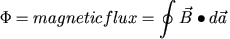A# magnetic flux (Φ)

Magnetic flux (Φ) is a measure of the quantity of magnetism or magnetic field. Specifically, magnetic flux, a scalar quantity, is defined as the surface integral (i.e. the integral over an area) of the magnetic flux density B:The SI unit of magnetic flux is the weber, named in honor of the German physicist, Wilhelm Eduard Weber (1804–1891).

Magnetic flux is a useful quantity in the formulation of Faraday's law of electromagnetic induction, and in discussing the physics of devices such as transformers and solenoids. In the case of an electric generator, where the magnetic field penetrates a rotating coil, the area used in defining the flux is the projection of the coil area onto the plane perpendicular to the magnetic field.

## Application of flux to Faraday's law

Michael Faraday's seminal experiments in electromagnetism led him to conclude that the magnitude of the electromotive force (emf) induced in a closed loop is proportional to the time rate of change of magnetic flux through the loop, i.e.,

ε = -C dΦ/dt

where ε is the instantaneous value of the induced emf, and C is a constant of proportionality. The negative sign in this equation is the basis for a sign convention governing ε.

When the flux is expressed in webers and the emf in volts, C = 1. Thus:

ε = -/dt

This emf is induced in a closed loop whether a conductor comprises the loop or not. It is present, for example, around any closed loop in space, through which the flux is changing.

If the flux passes through N identical turns of wire connected in series, instead of a single loop, the total induced emf is the sum of the emf's generated in the separate turns:

ε = -N /dt

This is Faraday's law of electromagnetic induction.